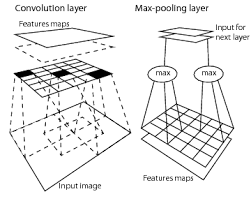# Pooling Layer in CNN (1)

Today I didn’t have the mood to continue my work on map merging of different cameras. So I read the paper from DeepMind of Learned Deformation Stability in Convolutional Neural Networks recommended by Wang Chen.

## 1. Convolution Operation

Convolution operation is typically denoted with an asterisk1: $$s(t)=(x*w)(t)$$ In Convolutional network terminology, the x is referred to as the input, and the w as the kernel. The output is sometimes referred to as the feature map.

Convolution leverages three ideas that help improve the ML system: sparse interactions, parameter sharing and equivariant representations. Moreover, convolution provides a means for working with inputs of variable size.

#### - Sparse interactions

Also called sparse connectivity or sparse weights, makes the kernel smaller than the input. By detecting small, meaningful features such as edges with kernels (tens or hundreds of pixels), we don’t need to process all the input pixels(millions), which means much fewer parameters.Sparse connectivity Parameter sharing

#### - Parameter sharing

Refers to using the same parameter for more than one function in a model, so a network has tied weights. This reduces the storage requirements of the model to k parameters.

#### - Equivariance to translation

But not to other transformations like scale or rotation change.

## 2. Pooling

In all cases, pooling helps to make the representation become approximately invariant to small translations of the input. Pooling over spatial regions produces invariance to translation, but if we pool over the outputs of separately parametrized convolutions, the features can learn which transformations to become invariant to.

Because pooling summarizes the responses over a whole neighborhood, it is possible to use fewer pooling units than detector units, by reporting summary statistics for pooling regions spaced k pixels apart rather than 1 pixel apart.

This article tries to analyze the relationship between the pooling layers and deformation stability in CNN based on the paper Learned Deformation Stability in Convolutional Neural Networks.

The paper tries to address the following questions: - Does pooling have an effect on the learned deformation stability?

• Is deformation stability achieved in the absence of pooling?

• How can deformation stability be achieved in the absence of pooling?

Traditional way of thinking pooling layer is that it is useful in two reasons:

1. By eliminating non-maximal (for max-pooling), it reduces computation for upper layers.
2. It porvides a form of translation invariance. Imagine cascading a max-pooling layer with a convolutional layer. There are 8 directions in which one can translate the input image by a single pixel. If max-pooling is done over a $2\times2$ region, 3 out of these 8 possible configurations will produce exactly the same output at the conv layer. For $3\times3$ windows, this jumps to $\frac{5}{8}$.

Then the author first defines the invariance to transformations of an input X that do not affect the output Y:

$$P(Y|\tau(X))=P(Y|X) \forall \tau \in T$$

Then he defines the measurement of sensitivity to deformation to evaluate this invariance.

For a representation r mapping from input image to some layer of a CNN, the measurement is:

$$\frac{dcos(r(x), r(\tau(x))}{median(dcos(r(x), r(y)))}$$ where dcos is the cosine distance. That is we normalize distances by the median distance between randomly selected images from the original dataset.

1. Ian Goodfellow, Yoshua Bengio, and Aaron Courville, Deep Learning, 2016. ^